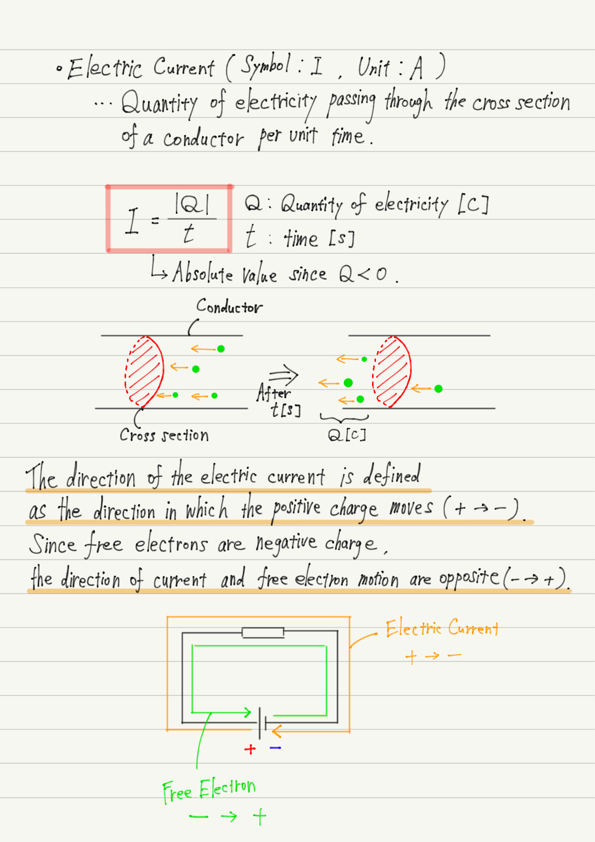# Electric Current

In this lecture, you will learn about electric currents. An electric current is a flow of charged particles.

Although electric current can flow in an electrolyte solution, high school physics only deals with current flowing through solids, so from now on we will limit our discussion to solids.

## Direction of electric current flow and free electron movement

Do you know in which direction current flows in an electric circuit?

Naturally, the answer is, “It flows from the positive to the negative electrode of the battery.”

Have you ever actually checked the direction of current flow?

Of course not, right? Because electric current is invisible!

What is the evidence that current flows from the cathode to the anode, even though no one has ever seen it before?

In fact, there is no evidence for this. It is simply “Because Ampère has decided so”.

Ampère knew that “something” was flowing through the conductors, but did not know what it was. So he decided, “Let’s assume that the electric current is flowing from the positive to the negative electrode of the battery.”

The direction of the current determined by Ampère was widely accepted throughout the world, but the situation changed drastically in the 20th century. At last we know what the current is. As we already know, the current flowing through a conductor is really electrons (Free Electrons)!

Although it is great that we now know what an electric current is, there is one annoying thing that has happened. That is that electrons have negative electricity.

Since free electrons have a negative charge, they will flow toward the positive electrode of the battery due to electrostatic forces. This is the opposite of the orientation Ampère determined! However, the direction of the current has not been corrected.

In summary, “the direction of current is from the positive to the negative pole” and “the direction of free electron movement is from the negative to the positive pole”. It is complicated, but remember them separately like this.

## Relationship between magnitude of electric current and quantity of electricity

Last but not least, let us discuss the magnitude of the electric current flowing through the conductor. Imagine a stream of water. What do you mean by a strong flow of water?

Fast flowing water, large volume of water, etc.

Let us apply this image directly to electric current. In other words, a large electric current means a fast free electron flow or a large number of free electrons flowing.

How can we determine the magnitude of the electric current to reflect this image?

To do so, it is convenient to determine the magnitude of the electric current as “the amount of electricity passing through the cross-section of a conductor per unit time.
This is because the faster the flow of free electrons, the more electrons can pass through in a second, and the more free electrons, the more electricity can pass through. This is exactly the way the water flow was described earlier!

## Summary of this lecture## Next Time

The next lecture will be on Voltage.

https://www.yukimura-physics.com/entry/elemag-f06

error: Content is protected !!